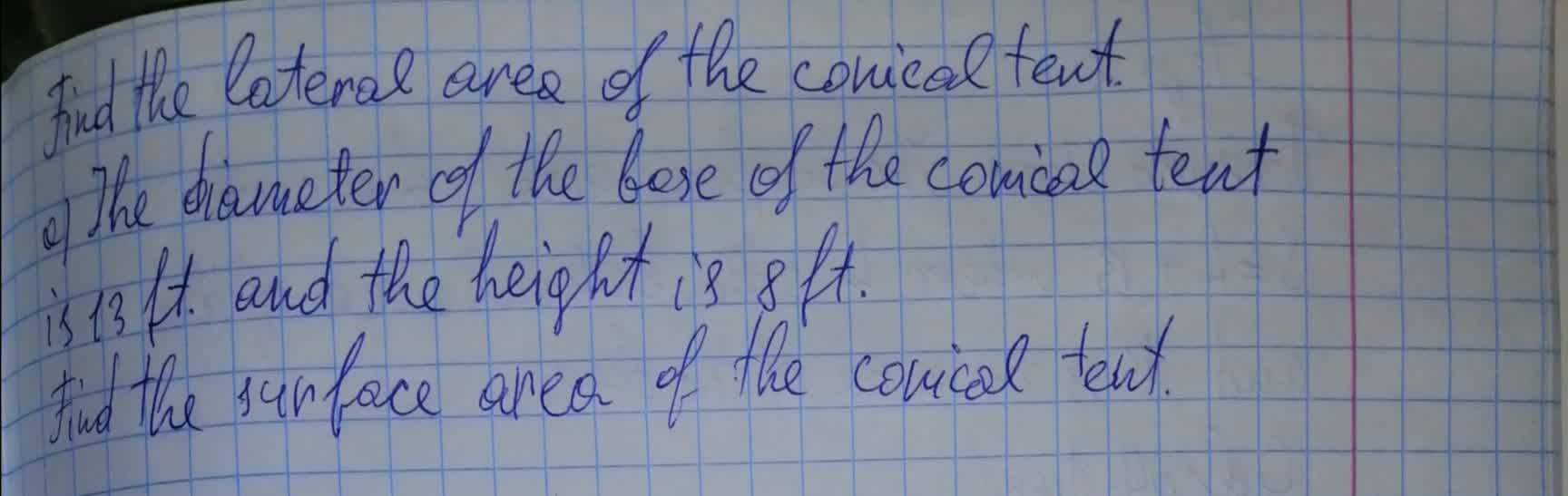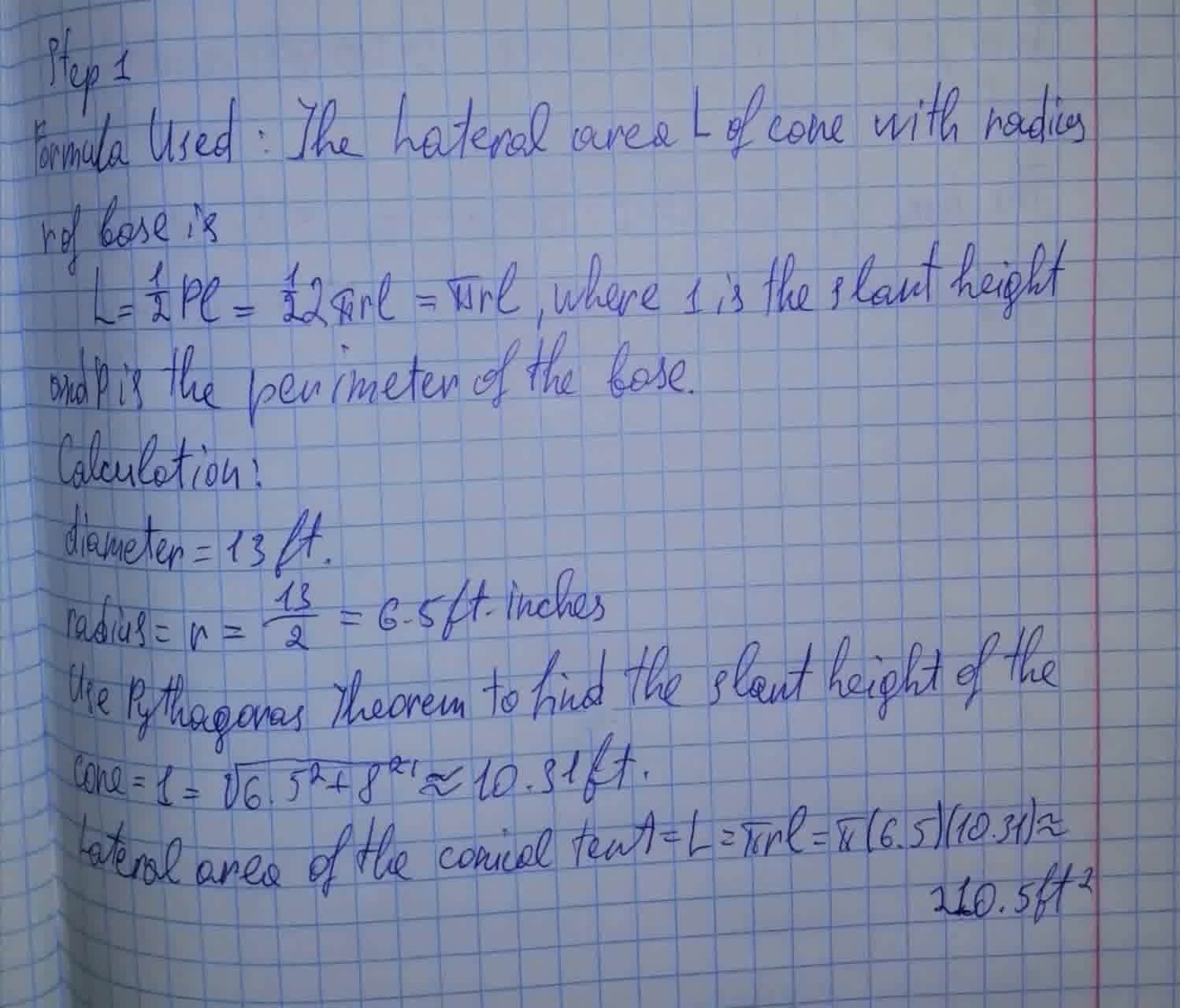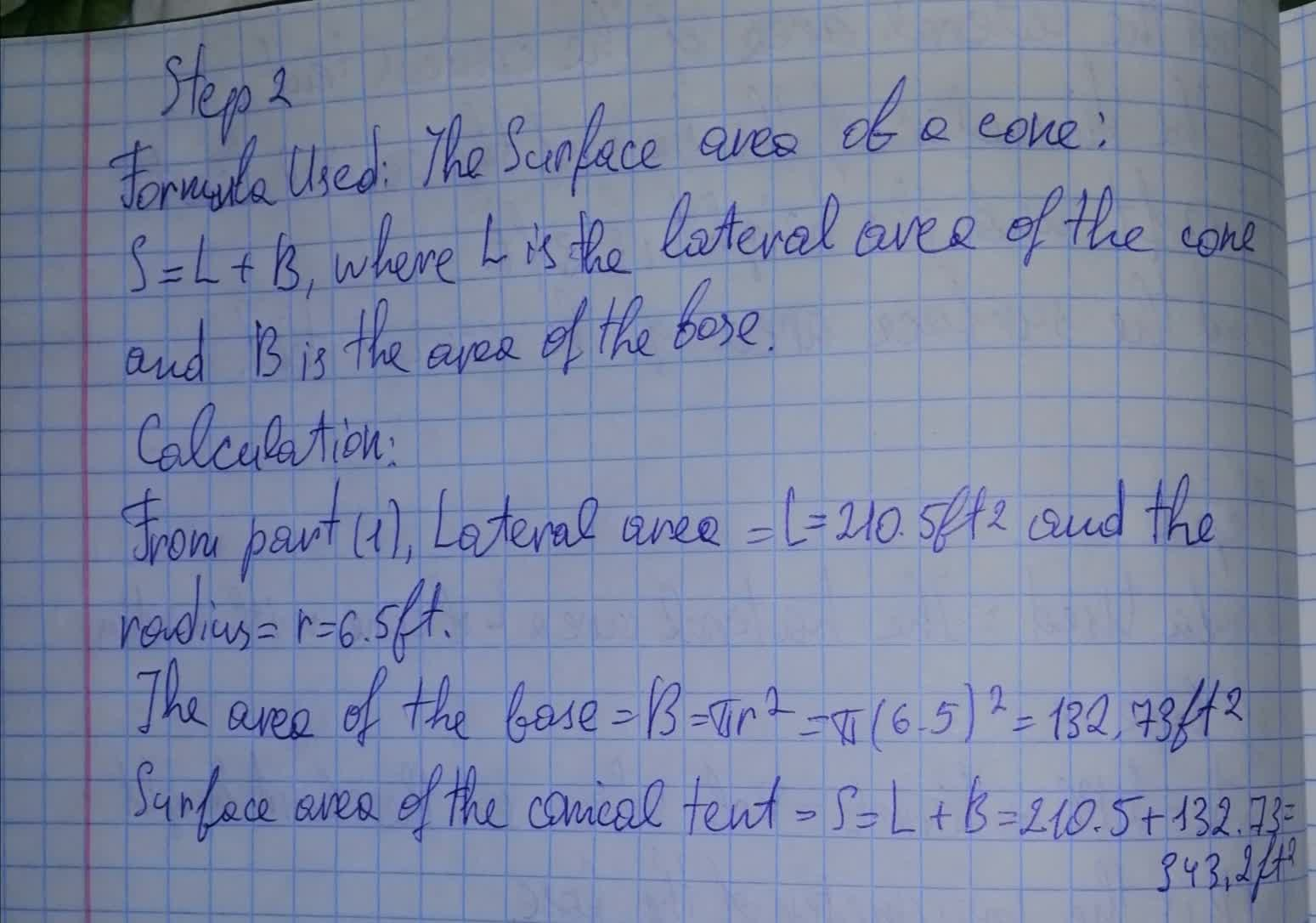# To determine: Find the lateral area of the conical tent.Anonym 2021-08-12 Answered
To determine: Find the lateral area of the conical tent.You can still ask an expert for help

• Questions are typically answered in as fast as 30 minutes

Solve your problem for the price of one coffee

• Math expert for every subject
• Pay only if we can solve iti1ziZ

The lateral area of the conical tent is###### Not exactly what you’re looking for?

Step 1

Formula used: The lateral area L of cone with radius r of base is

$L=\frac{1}{2}Pl=\frac{1}{2}2\pi rl=\pi rl$, where 1 is the slant height and P is the perimeter of the base

Calculation:

diameter=13 ft

radius$=r=\frac{13}{2}=6.5$ ft inches

Use Pythagoras Theorem to find the slant height of the cone $=1=\sqrt{{6.5}^{2}+{8}^{2}}\approx 10.31ft$

Lateral area of the conical tent $=L=\pi rl=\pi \left(6.5\right)\left(10.31\right)\approx 210.5f{t}^{2}$

Step 2

Formula used: The surface area of a cone:

S+L+B, where L is the lateral area of the cone and B is the area of the base.

Calculation:

From part(1), Lateral area

the area of the base

Surface area  of the conical tent